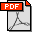Helmut Pottmann, Qixing Huang, Yongliang Yang, Shimin Hu: Geometry and Convergence Analysis of Algorithms for Registration of 3D Shapes. International Journal of Computer Vision 67(3): 277-296 (2006) Abstract: The computation of a rigid body transformation which optimally aligns a set of measurement points with a surface and related registration problems are studied from the viewpoint of geometry and optimization. We provide a convergence analysis for widely used registration algorithms such as ICP, using either closest points (Besl and McKay ) or tangent planes at closest points (Chen and Medioni ), and for a recently developed approach based on quadratic approximants of the squared distance function . ICP based on closest points exhibits local linear convergence only. Its counterpart which minimizes squared distances to the tangent planes at closest points is a Gauss-Newton iteration; it achieves local quadratic convergence for a zero residual problem and { if enhanced by regularization and step size control { comes close to quadratic convergence in many realistic scenarios. Quadratically convergent algorithms are based on the approach in . The theoretical results are supported by a number of experiments; there, we also compare the algorithms with respect to global convergence behavior, stability and running time. Bibtex: ```@article{phyh-gcar-06, author = {H. Pottmann and Q. Huang and Y. Yang and S. Hu}, title = {Geometry and Convergence Analysis of Algorithms for Registration of 3D Shapes}, journal = {Int. J. Comput. Vision}, volume = {67}, number = {3}, year = {2006}, issn = {0920-5691}, pages = {277--296}, } ```